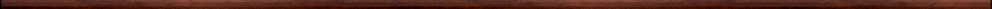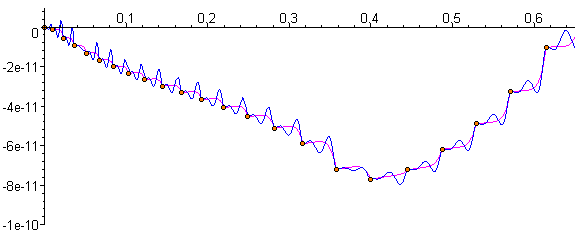Maple worksheets on interpolation for Runge-Kutta schemesNumerical methods topics:

They are all compatible with Classic Worksheet Maple 10.Interpolation for an order 5 Runge-Kutta scheme - RKinterp5a.mws

• The iterative construction of an interpolation scheme for an order 5 Prince-Dormand Runge-Kutta method.

An FSAL order 4-5 Runge-Kutta scheme together with interpolation - RKinterp5b.mws

• Derivation of Bogacki-Shampine order 4-5 Runge-Kutta scheme.
• The iterative construction of an interpolation scheme for the order 5 Bogacki-Shampine Runge-Kutta method

Interpolation for a Sharp-Verner (1994) order 6 Runge-Kutta scheme - RKinterp6a.mws

• The iterative construction of an interpolation scheme for a (1994) order 6 Sharp-Verner Runge-Kutta method.

Interpolation for a Verner (2004) order 6 Runge-Kutta scheme - RKinterp6b.mws

• The iterative construction of an interpolation scheme for a (2004) order 6 Verner Runge-Kutta method.

Interpolation for a Prince-Dormand order 6 Runge-Kutta scheme - RKinterp6c.mws

• The iterative construction of an interpolation scheme for an order 6 Prince-Dormand Runge-Kutta method.

Interpolation for a Verner (1991) order 6 Runge-Kutta scheme - RKinterp6d.mws

• The iterative construction of an interpolation scheme for a (1991) order 6 Verner Runge-Kutta method.

Interpolation for Verner's "most efficient" order 6 Runge-Kutta scheme - RKinterp6e.mws

• The iterative construction of an interpolation scheme for Verner's "most efficient" order 6 Runge-Kutta method.

Interpolation for an Enright (Verner formula) order 7 Runge-Kutta scheme - RKinterp7a.mws

• The iterative construction of an interpolation scheme for the Runge-Kutta method.

Interpolation for a "most robust" order 7 Runge-Kutta scheme - RKinterp7b.mws

• The iterative construction of an interpolation scheme for the "most robust" Runge-Kutta method.

Interpolation for a Sharp-Verner order 7 Runge-Kutta scheme - RKinterp7c.mws

• The iterative construction of an interpolation scheme for a Sharp-Verner order 7 Runge-Kutta method.

Interpolation for Maple's order 8 Runge-Kutta scheme - RKinterp8a.mws

• The iterative construction of an interpolation scheme for the order 8 Runge-Kutta method taken from Maple's "dverk78" scheme.

Interpolation for an Enright (Verner formula) order 8 Runge-Kutta scheme - RKinterp8b.mws

• The iterative construction of an interpolation scheme for the Verner formula Runge-Kutta method.

Interpolation for a Prince-Dormand order 8 Runge-Kutta scheme - RKinterp8c.mws

• The iterative construction of an interpolation scheme for an order 8 Prince-Dormand Runge-Kutta method.

Interpolation for Verner's "most robust" order 8 Runge-Kutta scheme - RKinterp8d.mws

• The iterative construction of an interpolation scheme for Verner's "most robust" order 8 Runge-Kutta method.

Interpolation for Verner's "most efficient" order 8 Runge-Kutta scheme - RKinterp8e.mws

• The iterative construction of an interpolation scheme for Verner's "most efficient" order 8 Runge-Kutta method.

Interpolation for Verner's "most robust" order 9 Runge-Kutta scheme - RKinterp9a.mws

• The iterative construction of an interpolation scheme for Verner's order 9 "most robust" Runge-Kutta method.

Interpolation for Tsitouras' order 9 Runge-Kutta scheme - RKinterp9b.mws

• The iterative construction of an interpolation scheme for Tsitouras' order 9 Runge-Kutta method.

Interpolation for Sharp's order 9 Runge-Kutta scheme - RKinterp9c.mws

• The iterative construction of an interpolation scheme for Sharp's order 9 Runge-Kutta method.

Top of page

Main index